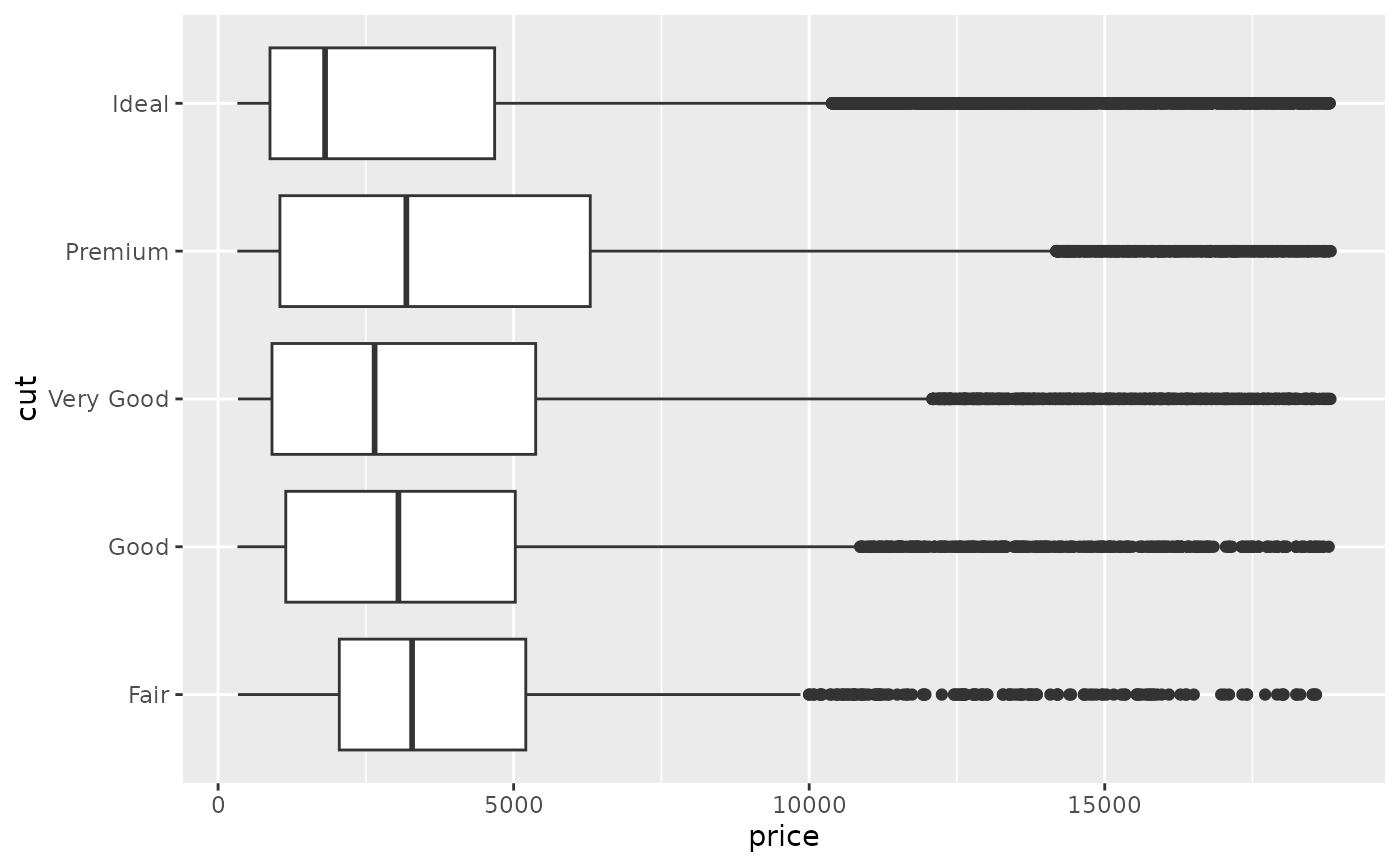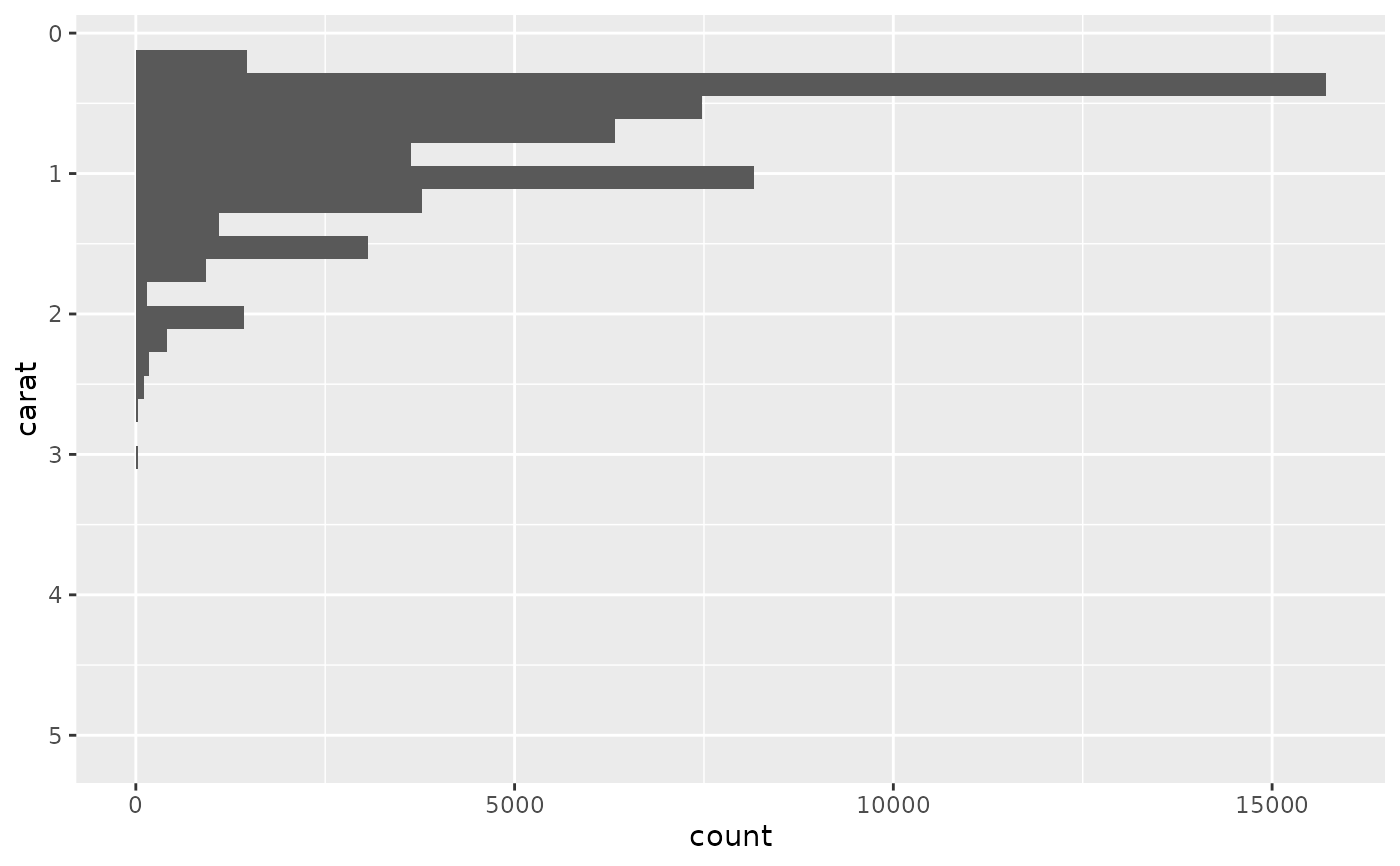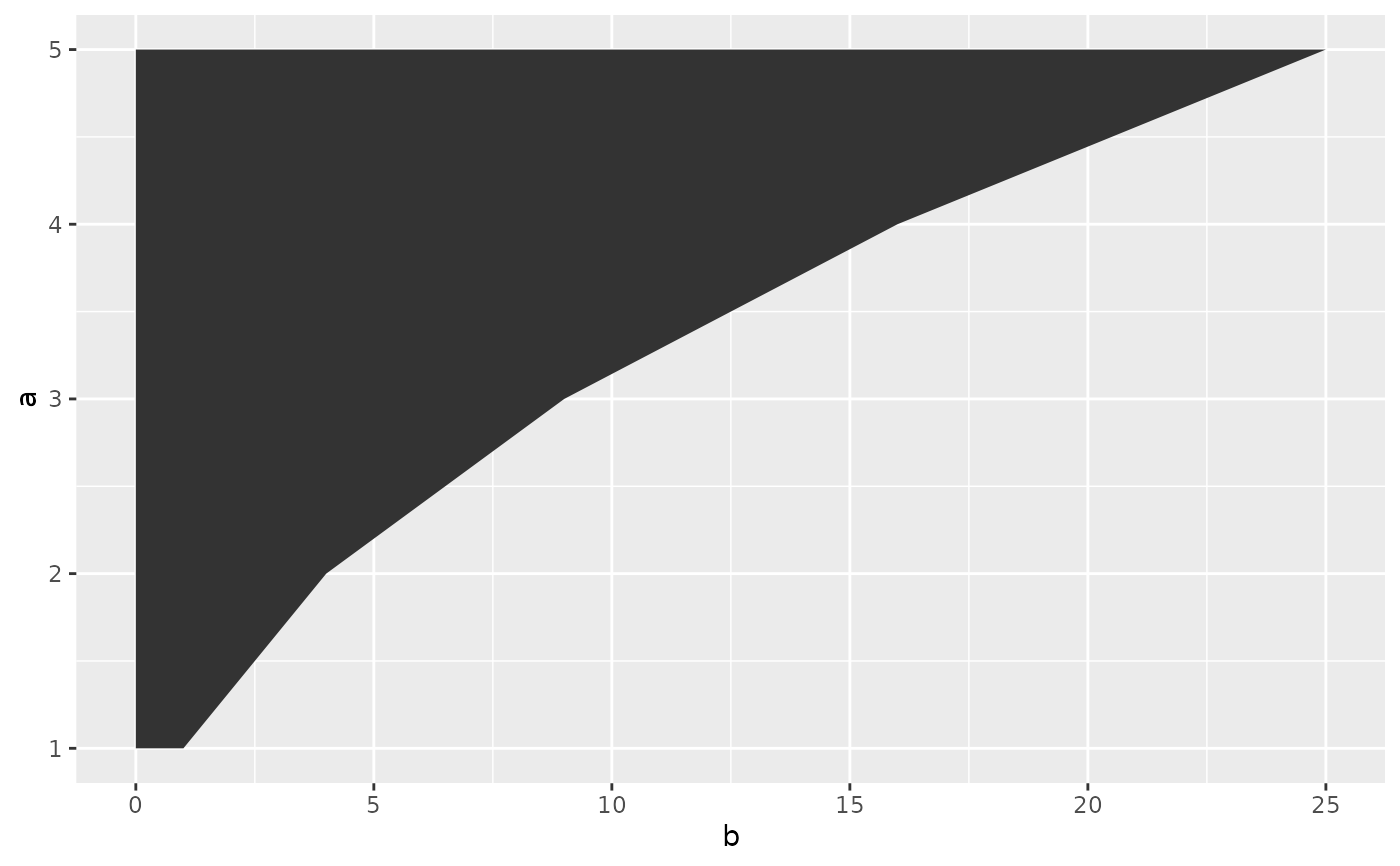This function is superseded because in many cases, coord_flip() can easily be replaced by swapping the x and y aesthetics, or optionally setting the orientation argument in geom and stat layers.

coord_flip() is useful for geoms and statistics that do not support the orientation setting, and converting the display of y conditional on x, to x conditional on y.

## Usage

coord_flip(xlim = NULL, ylim = NULL, expand = TRUE, clip = "on")

## Arguments

xlim, ylim

Limits for the x and y axes.

expand

If TRUE, the default, adds a small expansion factor to the limits to ensure that data and axes don't overlap. If FALSE, limits are taken exactly from the data or xlim/ylim.

clip

Should drawing be clipped to the extent of the plot panel? A setting of "on" (the default) means yes, and a setting of "off" means no. In most cases, the default of "on" should not be changed, as setting clip = "off" can cause unexpected results. It allows drawing of data points anywhere on the plot, including in the plot margins. If limits are set via xlim and ylim and some data points fall outside those limits, then those data points may show up in places such as the axes, the legend, the plot title, or the plot margins.

## Examples

# The preferred method of creating horizontal instead of vertical boxplots
ggplot(diamonds, aes(price, cut)) +
geom_boxplot()# Using coord_flip() to make the same plot
ggplot(diamonds, aes(cut, price)) +
geom_boxplot() +
coord_flip()# With swapped aesthetics, the y-scale controls the left axis
ggplot(diamonds, aes(y = carat)) +
geom_histogram() +
scale_y_reverse()
#> stat_bin() using bins = 30. Pick better value with binwidth.# In coord_flip(), the x-scale controls the left axis
ggplot(diamonds, aes(carat)) +
geom_histogram() +
coord_flip() +
scale_x_reverse()
#> stat_bin() using bins = 30. Pick better value with binwidth.# In line and area plots, swapped aesthetics require an explicit orientation
df <- data.frame(a = 1:5, b = (1:5) ^ 2)
ggplot(df, aes(b, a)) +
geom_area(orientation = "y")# The same plot with coord_flip()
ggplot(df, aes(a, b)) +
geom_area() +
coord_flip()